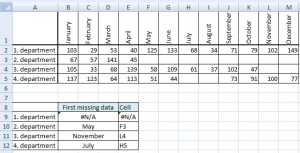# Address of the first empty cell in a row

In some cases it is useful to know the address of the first empty cell in a row. This is a way to do this without macros. Write the following formula and press CTRL+SHIFT+ENTER. Excel adds braces { } and saves it as array formula.

## Example 1` {=ADDRESS(3,COLUMN(INDEX(B3:F3,1,MATCH(TRUE,LEN(B3:F3)=0,0))))}`

## Example 2When (in which month) the department interrupted filling data? We are searching for the first blank cell in the row of department 1, 2, 3 and 4. Formula for the first department:

`{=INDEX(\$B\$1:\$M\$1,1,MATCH(TRUE,LEN(B2:M2)=0,0))}`

Note: Value #N/A means that there is no missing data.

And which cell is it?

`{=ADDRESS(ROW(B2),COLUMN(INDEX(B2:M2,1,MATCH(TRUE,LEN(B2:M2)=0,0))),4)}`

1.Can you please release me the solution to know the first non-empty cell in the row, when parsing from the right of the sheet (not from the left)?Thanks in advance
Antonio

2.Can I use some VBA macro instead of this complicated formula?

3.What if you wanted to know the first non-empty cell in the row, only parsing from the right, not the left. How would you do that?

4.This worked perfect… thanks!